# SAT Subject Math Level 1 Practice Test 12

### Test Information20 questions24 minutes

Take more free SAT math 1&2 subject practice tests available from cracksat.net.

1. A car travels a steady speed of x miles per hour. How many hours, in terms of x, will it take to drive 540 miles?

A. 540x
B. 540 + x
C.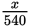D.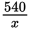E. x - 540

2.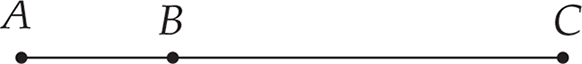In the segment shown in Figure above, the length of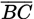is three more than twice the length of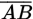. If the length of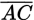is 27 cm, what is the length of?

A. 8 cm
B. 9 cm
C. 10 cm
D. 12 cm
E. 15 cm

3. If f(x) = x2 - 10 and g(x)= 4x + 3, what is f(g(2))?

A. -24
B. -21
C. 12
D. 27
E. 111

4. Which graph below represents the solution to the following system of inequalities?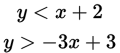A.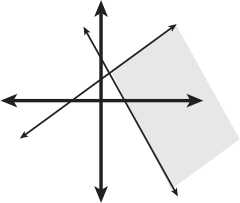B.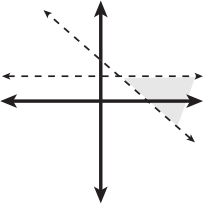C.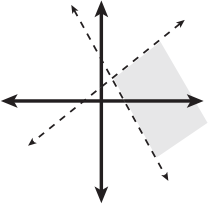D.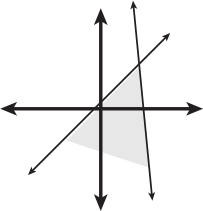E.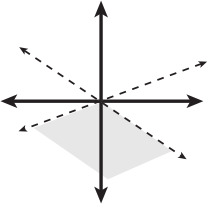5.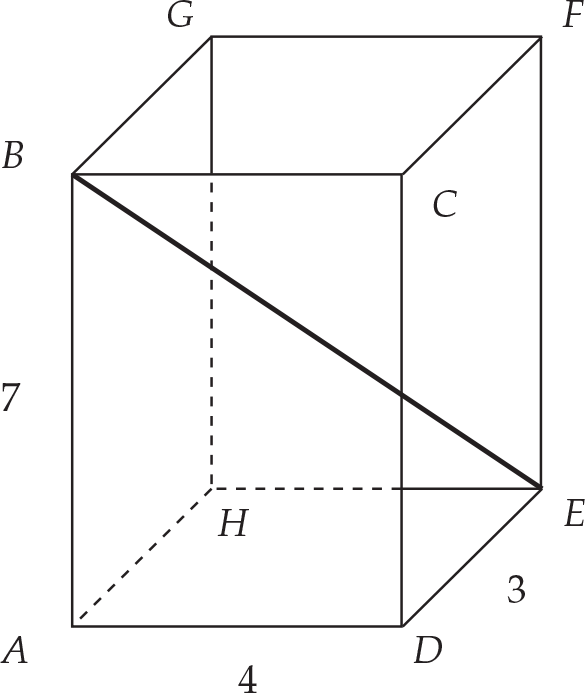Figure3

In the rectangular solid shown in Figure 3, what is the length of diagonal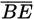, to the nearest tenth?

A. 7.07
B. 8.6
C. 10
D. 13.60
E. 25

6.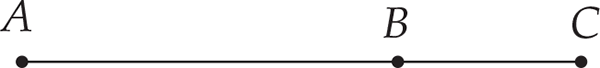Figure4

In the segment shown in Figure 4, the ratio of the lengths oftois 5:8. If x represents AB, what is the midpointofin terms of x?

A. 4x
B.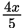C.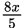D.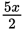E.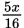7. Let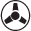be defined asab =a2 + ba - 16b ÷ a.What is the value of 83?

A. -2.67
B. 5
C. 34
D. 46
E. 82

8.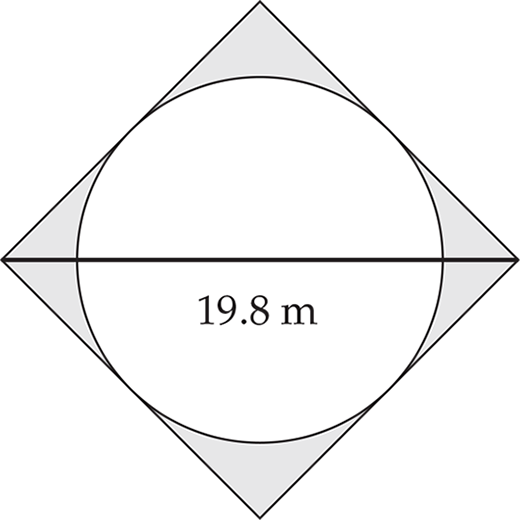Figure5

In Figure5, a circle is inscribed in a square. What is the area of the shaded portion, to the nearest hundredth?

A. 10.52 m2
B. 21.03 m2
C. 22.6 m2
D. 42.06 m2
E. 84.13 m2

9.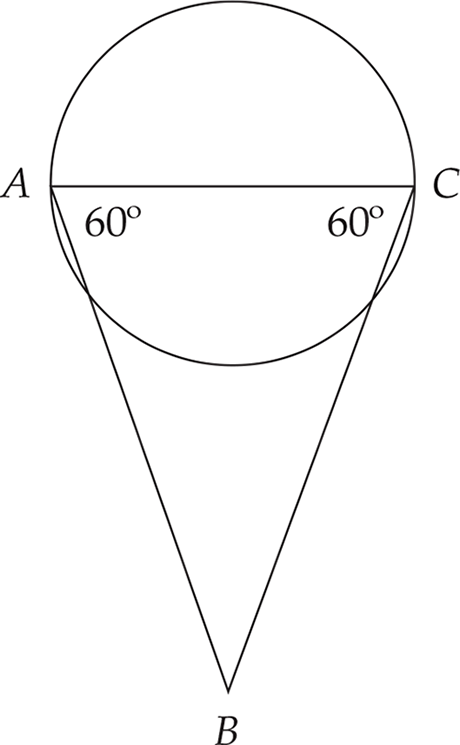Figure6

In Figure6, If the area of the circle is 64πsquare units, what is the area of triangle ABC to the nearest hundredth?

A. 55.43
B. 110.85
C. 128
D. 221.7
E. 443.41

10.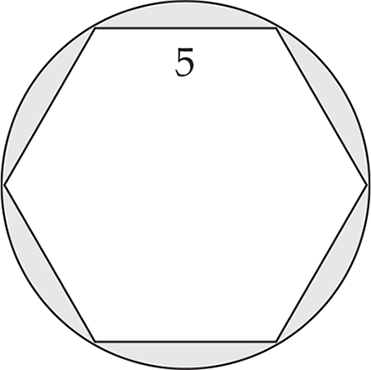Figure7

In Figure7, a regular hexagon of side length 5 cm is inscribed in a circle. What percentage of the circle is shaded, to the nearest tenth?

A. 13.6%
B. 17.3%
C. 78.5%
D. 82.7%
E. 86.4%

11. A dog is chained on a 6-foot leash, fastened to the corner of a rectangular building. About how much area does the dog have to move in?

A. 27 ft2
B. 36 ft2
C. 56.55 ft2
D. 84.82 ft2
E. 113.10 ft2

12. What is the domain of the function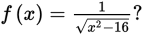A. All the real numbers
B. x < -4 orx > 4
C. x ≥ 4
D. x > 8
E. x < -8 orx > 8

13. Given the following stem-and-leaf plot and the statements shown below the plot,which of the statements are true?

The number of customers in line for an attraction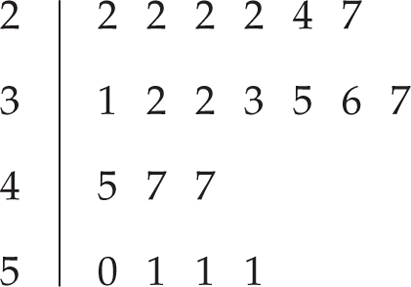where 3 |6 means 36 customers

The mode is equal to the median.

The median is less than the mean.

The mean is 33.

A. I only
B. II only
C. III only
D. I and II only
E. II and III only

14.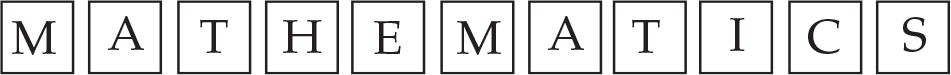What is the probability of picking an M card at random, without replacement, and the n an A card, at random, without looking?

A.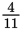B.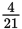C.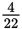D.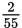E.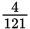15.What is the probability of picking an S card at random, without replacement, and the n NOT picking a T card, at random, without looking?

A.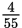B.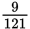C.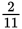D.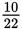E.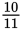16.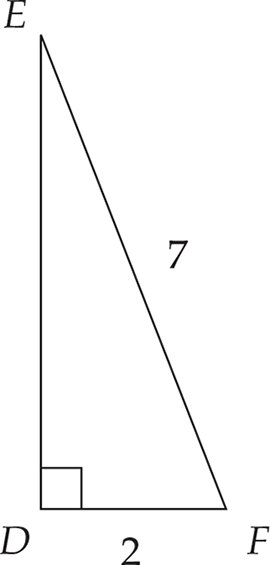Figure8

In right triangle EDF in Figure 8, the length of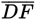is 2 cm, and the length of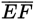is 7 cm. What is the measure of ∠EFD, to the nearest hundredth of a degree?

A. 15.95°
B. 16.6°
C. 73.40°
D. 90°
E. 99.9°

17. If f(x) = x2 - 7, the nf(a - 3) is

A. a2- 6a - 16
B. a2- 10
C. a2 +21
D. a2- 6a + 2
E. 2a - 13

18. Given pointA(-3,-8), If the midpoint of segment AB is(1,-5), what are the coordinates of point B?

A. (5,-2)
B. (4,-2)
C. (-1,-6.5)
D. (-2,-2)
E. (-1,-1.5)

19. The area of the triangle with coordinates (1,2), (5,5), and (k,2) is 15square units. What is a possible value for k?

A. -10
B. -9
C. -5
D. 5
E. 6

20.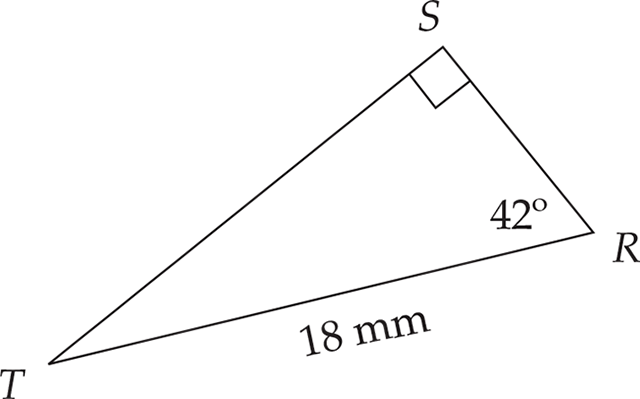Given right triangle RST in Figure above, what is the length of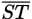, to the nearest hundredth?

A. 12.04 mm
B. 13.38 mm
C. 16.21 mm
D. 24.22 mm
E. 26.90 mm

﻿